# Markov chain modeling¶

In this tutorial, we implement a Markov chain model that allows for different orders. We demonstrate the model on human navigation data derived from Wikispeedia.

## Markov chain model¶

First, we implement the model

In :
# further implementation can be found at https://github.com/psinger/PathTools

from __future__ import division

import itertools
from scipy.sparse import csr_matrix
from scipy.special import gammaln
from scipy.stats import chi2
from collections import defaultdict
from sklearn.preprocessing import normalize
import numpy as np

class MarkovChain():
"""
Markov Chain Model
"""

# dummy constant for our reset state
RESET_STATE = "-1"

def __init__(self, order=1, reset=True):
"""
Constructor for class MarkovChain

Args:
order: Order of Markov Chain model
reset: Boolean for using additional reset states
"""
self.order = order
self.reset = reset

def fit(self,sequences):
"""
Function for fitting the Markov Chain model given data

Args:
sequences: Data of sequences, list of lists
"""

# first, we derive all basic states from given sequences
states = set(itertools.chain.from_iterable(sequences))
self.state_count = len(states)
if self.reset:
self.state_count += 1

# dictionary of dictionaries for counting transitions between states
transitions = defaultdict(lambda : defaultdict(float))

# remember all start and target states that we observe
# this is just necessary for memory saving reasons
start_states = set()
target_states = set()

# iterate through sequences
for seq in sequences:
i = 0
# Prepend and append correct amount of reset states if flag set
if self.reset:
seq = self.order*[self.RESET_STATE] + seq + [self.RESET_STATE]
# iterate through elements of a sequence
for j in xrange(self.order, len(seq)):
# start state based on order
elemA = tuple(seq[i:j])
# target state based on order
elemB = tuple(seq[j-self.order+1:j+1])
i += 1

# increase transition count
transitions[elemA][elemB] += 1

# remember start and target states

# build vocabularies for mapping states to indices
self.start_vocab = dict((v,k) for k,v in enumerate(start_states))
self.target_vocab = dict((v,k) for k,v in enumerate(target_states))

# transform transition dictionary of dictionaries to sparse csr_matrix
i_indices = []
j_indices = []
values = []

for s,ts in transitions.iteritems():
for t,c in ts.iteritems():
i_indices.append(self.start_vocab[s])
j_indices.append(self.target_vocab[t])
values.append(c)
shape = (len(start_states), len(target_states))
self.transitions = csr_matrix((values, (i_indices, j_indices)),
shape=shape)

#print "fit done"

def loglikelihood(self):
"""
Returns the loglikelihood given fitted model

Returns:
loglikelihood
"""

# get mle by row-normalizing transition matrix
self.mle = normalize(self.transitions,norm="l1",axis=1)
# log mle
mle_log = self.mle.copy()
mle_log.data = np.log(mle_log.data)
return self.transitions.multiply(mle_log).sum()

@staticmethod
def lrt(ln, pn, lt, pt):
"""
Performs a likelihood ratio test given a null and alternative model

Args:
ln: loglikelihood of null model
pn: nr. of parameters of null model
lt: loglikelihood of alternative model
pt: nr. of parameters of alternative model
Returns:
lr: log-likelihood ratio
p_val: p value
"""

# likelihood ratio
lr = float(-2*(ln-lt))
# degrees of freedom
dof = float(pt - pn)
# chi2 test
p_val = chi2.sf(lr,dof)
return lr, p_val

def aic(self):
"""
Determines the AIC given fitted model

Returns:
aic
"""
ll = self.loglikelihood()
# parameter count of model
para = self.state_count**self.order*(self.state_count-1)
return 2*para - 2*ll

def bic(self):
"""
Determines the BIC given fitted model

Returns:
bic
"""
ll = self.loglikelihood()
# parameter count of model
para = self.state_count**self.order*(self.state_count-1)
return para*np.log(self.transitions.sum()) - 2*ll

def evidence(self, prior=1.):
"""
Determines Bayesian evidence given fitted model

Args:
prior: Dirichlet prior parameter (symmetric)
Returns:
evidence
"""

i_dim, j_dim = self.transitions.shape

# elegantly calculate evidence
evidence = 0
evidence += i_dim * gammaln(self.state_count*prior)
evidence -= gammaln(self.transitions.sum(axis=1)+self.state_count*prior).sum()
evidence += gammaln(self.transitions.data+prior).sum()
evidence -= len(self.transitions.data) * gammaln(prior)

return evidence


## Slides example¶

For a first demonstration, we present the example from the slides consisting of blue and yellow states.

### Data¶

In :
sequences = [["b","b","b","y","y","b","y","b","b","b","b"]]


### Fitting¶

We fit both a first and second order model and print the transition matrices.

In :
print "First-order model"
mc1 = MarkovChain(reset=True, order=1)
mc1.fit(sequences)
print mc1.transitions.todense()

print "---------"
print "Second-order model"
mc2 = MarkovChain(reset=True, order=2)
mc2.fit(sequences)
print mc2.transitions.todense()

First-order model
[[ 1.  2.  0.]
[ 2.  5.  1.]
[ 0.  1.  0.]]
---------
Second-order model
[[ 0.  0.  0.  0.  1.  1.]
[ 1.  3.  0.  1.  0.  0.]
[ 0.  1.  0.  0.  0.  0.]
[ 0.  0.  1.  0.  0.  0.]
[ 1.  1.  0.  0.  0.  0.]
[ 0.  0.  0.  0.  1.  0.]]


### Model selection¶

Next, let us compare the two models.

In :
print "First-order model"
print "Log-likelihood:", mc1.loglikelihood()
print "Evidence:", mc1.evidence()
print "AIC:", mc1.aic()
print "BIC:", mc1.bic()

print "---------"
print "Second-order model"

print "Log-likelihood:", mc2.loglikelihood()
print "Evidence:", mc2.evidence()
print "AIC:", mc2.aic()
print "BIC:", mc2.bic()

First-order model
Log-likelihood: -9.11159091503
Evidence: -13.4304361395
AIC: 30.2231818301
BIC: 33.1326217288
---------
Second-order model
Log-likelihood: -7.52394141841
Evidence: -14.3059048769
AIC: 51.0478828368
BIC: 59.776202533


The simpler, first-order model is to be preferred.

## Synthetic example¶

Now, let us generate synthetic data with given memory. We always start with the state "A" and add additional "B" states based on a given memory. Then, we repeat this process 1000 times. For example, for a simple Markovian first-order process, we would produce "ABABABAB...". For a third-order process we would produce "ABBBABBB...". Then, the model comparison criteria correctly determine corresponding Markov chain model as the most appropriate ones.

In :
%matplotlib inline
import matplotlib.pyplot as plt

for o in xrange(1,5):
sample = [(["A"]+["B"]*o)*1000]

loglikelihoods = []
aics = []
bics = []
evidences = []

max_order = 5
fig = plt.figure()

for i in xrange(1,max_order+1):
mc = MarkovChain(reset=True, order=i)
mc.fit(sample)
loglikelihoods.append(mc.loglikelihood())
aics.append(mc.aic())
bics.append(mc.bic())
evidences.append(mc.evidence())

# let us just plot the evidences here, the other criteria confirm these results
ax.plot(xrange(1,max_order+1), evidences)
max_index = evidences.index(max(evidences))
ax.plot((xrange(1,max_order+1))[max_index], evidences[max_index], 'rD', clip_on = False)
ax.set_title(sample[:o+1])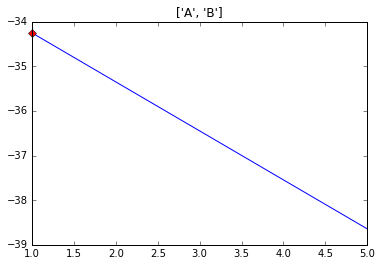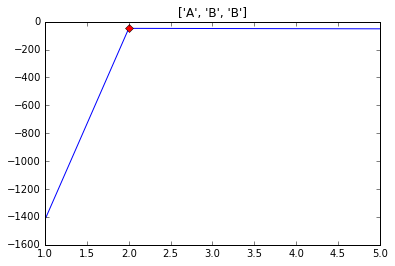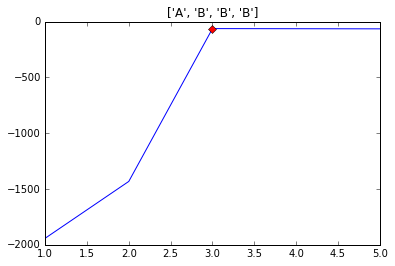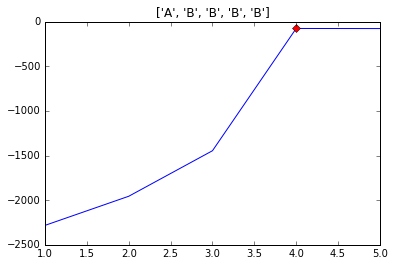As expected, the modeling selection techniques also indicate those orders as the most plausible after which the data has been generated.

## Wikispeedia human Web navigation example¶

We now focus on an example of human Web navigation.

### Data¶

We load our data into a list of lists where each element corresponds to a sequence.

In :
import csv
sequences = []

# from https://snap.stanford.edu/data/wikispeedia.html
for line in csv.reader((row for row in open("data/paths_finished.tsv") if not row.startswith('#')), delimiter='\t'):
if len(line) == 0:
continue
# for simplicity, let us remove back clicks
seq = line.split(";")
# for simplicity, let us remove back clicks
seq = [x for x in seq if x != "<"]
sequences.append(seq)

In :
print sequences[:10]

[['14th_century', '15th_century', '16th_century', 'Pacific_Ocean', 'Atlantic_Ocean', 'Accra', 'Africa', 'Atlantic_slave_trade', 'African_slave_trade'], ['14th_century', 'Europe', 'Africa', 'Atlantic_slave_trade', 'African_slave_trade'], ['14th_century', 'Niger', 'Nigeria', 'British_Empire', 'Slavery', 'Africa', 'Atlantic_slave_trade', 'African_slave_trade'], ['14th_century', 'Renaissance', 'Ancient_Greece', 'Greece'], ['14th_century', 'Italy', 'Roman_Catholic_Church', 'HIV', 'Ronald_Reagan', 'President_of_the_United_States', 'John_F._Kennedy'], ['14th_century', 'Europe', 'North_America', 'United_States', 'President_of_the_United_States', 'John_F._Kennedy'], ['14th_century', 'China', 'Gunpowder', 'Fire'], ['14th_century', 'Time', 'Isaac_Newton', 'Light', 'Color', 'Rainbow'], ['14th_century', 'Time', 'Light', 'Rainbow'], ['14th_century', '15th_century', 'Plato', 'Nature', 'Ultraviolet', 'Color', 'Rainbow']]

In :
len(sequences)

Out:
51318

### Fitting¶

We now fit the Markov chain model to the data. We vary the order from 1 to 5. Additionally, we determine all model comparison techniques and store them for each order at hand.

In :
loglikelihoods = []
aics = []
bics = []
evidences = []
lrts = []

max_order = 5

for i in xrange(1,max_order+1):
mc = MarkovChain(reset=True, order=i)
mc.fit(sequences)
loglikelihoods.append(mc.loglikelihood())
aics.append(mc.aic())
bics.append(mc.bic())
evidences.append(mc.evidence())

for i, ln in enumerate(loglikelihoods):
for j, lt in enumerate(loglikelihoods[i+1:]):
lrt = mc.lrt(ln, mc.state_count**(i+1)*(mc.state_count-1), lt, mc.state_count**(i+1+j+1)*(mc.state_count-1))
lrts.append([str(i+1)+" vs. "+str(i+1+j+1),lrt,lrt])


### Model Selection¶

Next, we select the most appropriate model.

#### Likelihood ratio test¶

Perform likelihood ratio test between all fits

In :
print "orders | ", "likelihood ratio | ", "p-value"
for x in lrts:
print " | ".join([str(k) for k in x])

orders |  likelihood ratio |  p-value
1 vs. 2 | 862481.65175 | 1.0
1 vs. 3 | 1405620.38073 | 1.0
1 vs. 4 | 1538326.871 | 1.0
1 vs. 5 | 1558210.23636 | 1.0
2 vs. 3 | 543138.728976 | 1.0
2 vs. 4 | 675845.219245 | 1.0
2 vs. 5 | 695728.584614 | 1.0
3 vs. 4 | 132706.490269 | 1.0
3 vs. 5 | 152589.855638 | 1.0
4 vs. 5 | 19883.3653686 | 1.0


#### AIC, BIC and Evidence¶

Now, we determine the AIC, BIC and Evidence for all fits. Additionally, we plot the simple log-likelihood of the fits.

In :
f, ((ax1, ax2), (ax3, ax4)) = plt.subplots(2,2,figsize=(8,6))

x = xrange(1,max_order+1)

ax1.plot(x, loglikelihoods)
max_index = loglikelihoods.index(max(loglikelihoods))
ax1.plot(x[max_index], loglikelihoods[max_index], 'rD', clip_on = False)
ax1.set_ylabel("Log-likelihood")
ax2.plot(x, aics)
min_index = aics.index(min(aics))
ax2.plot(x[min_index], aics[min_index], 'rD', clip_on = False)
ax2.set_ylabel("AIC")
ax3.plot(x, bics)
min_index = bics.index(min(bics))
ax3.plot(x[min_index], bics[min_index], 'rD', clip_on = False)
ax3.set_ylabel("BIC")
ax4.plot(x, evidences)
max_index = evidences.index(max(evidences))
ax4.plot(x[max_index], evidences[max_index], 'rD', clip_on = False)
ax4.set_ylabel("Evidence")

plt.tight_layout()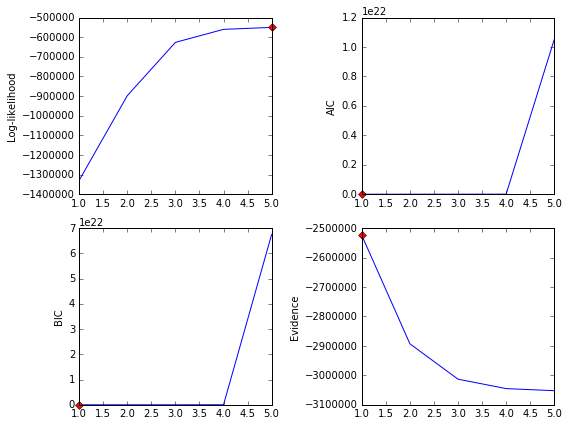#### Summary¶

All modeling comparison techniques indicate that a first-order Markov chain model sufficiently models given data. While higher order models always produce better fits due to nested models, their increasing number of necessary parameters does not fully justify their increasing complexity. However, with less states and/or more data, this might be different.